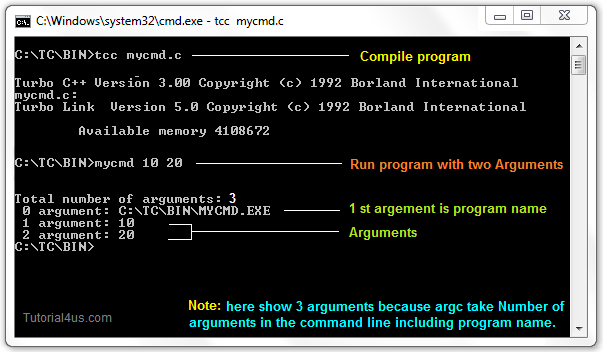# Command Line Argument in C

## Command Line Argument

If any input value is passed through command prompt at the time of running of program is known as command line argument. It is a concept to passing the arguments to the main() function by using command prompt.

## When Use Command Line Argument

When you need to developing an application for DOS operating system then in that case command line arguments are used. DOS operating system is a command interface operating system so by using command we execute the program. With the help of command line arguments we can create our own commands.

In Command line arguments application main() function will takes two arguments that is;

• argc
• argv

argc: argc is an integer type variable and it holds total number of arguments which is passed into main function. It take Number of arguments in the command line including program name.

argv[]: argv[] is a char* type variable, which holds actual arguments which is passed to main function.

### Compile and run CMD programs

Command line arguments are not compile and run like normal C programs, these programs are compile and run on command prompt. To Compile and Link Command Line Program we need TCC Command.

• First open command prompt
• For compile -> C:/TC/BIN>TCC mycmd.c
• For run -> C:/TC/BIN>mycmd 10 20
• Explanation: Here mycmd is your program file name and TCC is a Command. In "mycmd 10 20" statement we pass two arguments.

## Example of Command line argument

```#include<stdio.h>
#include<conio.h>

void main(int argc, char* argv[])
{
int i;
clrscr();
printf("Total number of arguments: %d",argc);
for(i=0;i< argc;i++)
{
printf("\n %d argument: %s",i,argv[i]);
getch();
}
}
```

## Output

```C:/TC/BIN>TCC mycmd.c
C:/TC/BIN>mycmd 10 20
Number of Arguments: 3
0 arguments c:/tc/bin/mycmd.exe
1 arguments: 10
2 arguments: 20
```

Note: In above output we passed two arguments but is show "Number of Arguments: 3" because argc take Number of arguments in the command line including program name. So here two arguments and one program name (mycmd.exe) total 3 arguments.## Example of Command line argument

```#include<stdio.h>
#include<conio.h>

void main(int argc, char* argv[])
{
clrscr();
printf("\n Program name  : %s \n", argv);
printf("1st arg  : %s \n", argv);
printf("2nd arg  : %s \n", argv);
printf("3rd arg  : %s \n", argv);
printf("4th arg  : %s \n", argv);
printf("5th arg  : %s \n", argv);
getch();
}
```

## Output

```C:/TC/BIN>TCC mycmd.c
C:/TC/BIN>mycmd this is a program
Program name : c:/tc/bin/mycmd.c
1st arg : this
2nd arg : is
3rd arg : a
4th arg : program
5th arg : (null)
```

Explanation: In the above example.

## Example

```argc      =   5
argv   =   "mycmd"
argv   =   "this"
argv   =   "is"
argv   =   "a"
argv   =   "program"
argv   =   NULL
```

### Why command line arguments program not directly run form TC IDE

Command line arguments related programs are not execute directly from TC IDE because arguments can not be passed.

## Edit Command Line Argument Program

To Edit the Command Line Argument Program use edit Command.

## Syntax

```C:/cprogram>edit mycmd.c
```
• Whenever the program is compiled and link we will get .exe file and that .exe file itself is command.
• In above example program name is mycmd.c so executable file is mycmd.exe and command name is mycmd.
• To load the application in to the memory we required to use program name and command name.

## Access data from outside of main

• argc and agrv are local variables to main function because those are the main function parameters.
• According to the storage classes of C argc and argv are auto variable to main function, so we can not extend the range of auto variable.
• By using argc and argv we can not access command from data outside of the main function.
• In implementation when we required to access command from data outside of the main function then use _argc, _argv variables.
• _argc and _argv are global variable which is declared in dos.h.

## Example

```#include<stdio.h>
#include<conio.h>
#include<dos.h>

void abc()
{
int i;
printf("data in abc:");
printf("\n Total no. of arguments: %d",_argc);
for (i=0;i< _argc;i++)
{
printf("\n %d argument: %s",i+1,_argv[i]);
}
}
void main(int argc, char*argv[])
{
int i;
clrscr();
printf("\n data in main:");
printf("\n total no. of arguments: %d",argc);
for(i=0;i< argc;i++)
{
printf("\n %d arguments:%s",i+1,argv[i]);
}
abc();
getch();
}
```